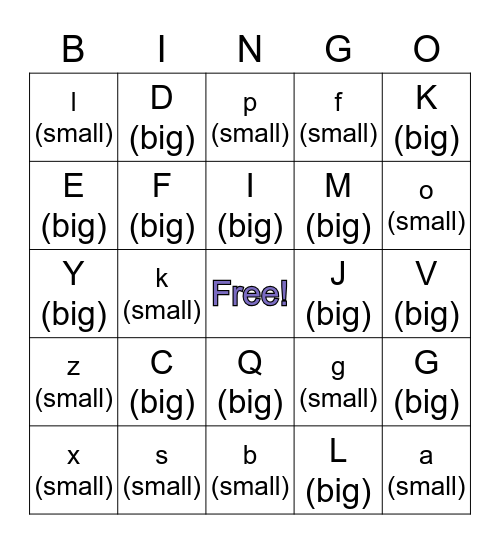# Big and Small LettersThis bingo card has a free space and 50 words: A (big), a (small), B (big), b (small), C (big), c (small), D (big), d (small), E (big), e (small), F (big), f (small), G (big), g (small), H (big), h (small), I (big), J (big), j (small), K (big), k (small), L (big), l (small), M (big), m (small), O (big), o (small), P (big), p (small), Q (big), q (small), R (big), r (small), S (big), s (small), T (big), t (small), U (big), u (small), V (big), v (small), W (big), w (small), X (big), x (small), Y (big), y (small), Z (big), z (small) and i (small).

## Play Online